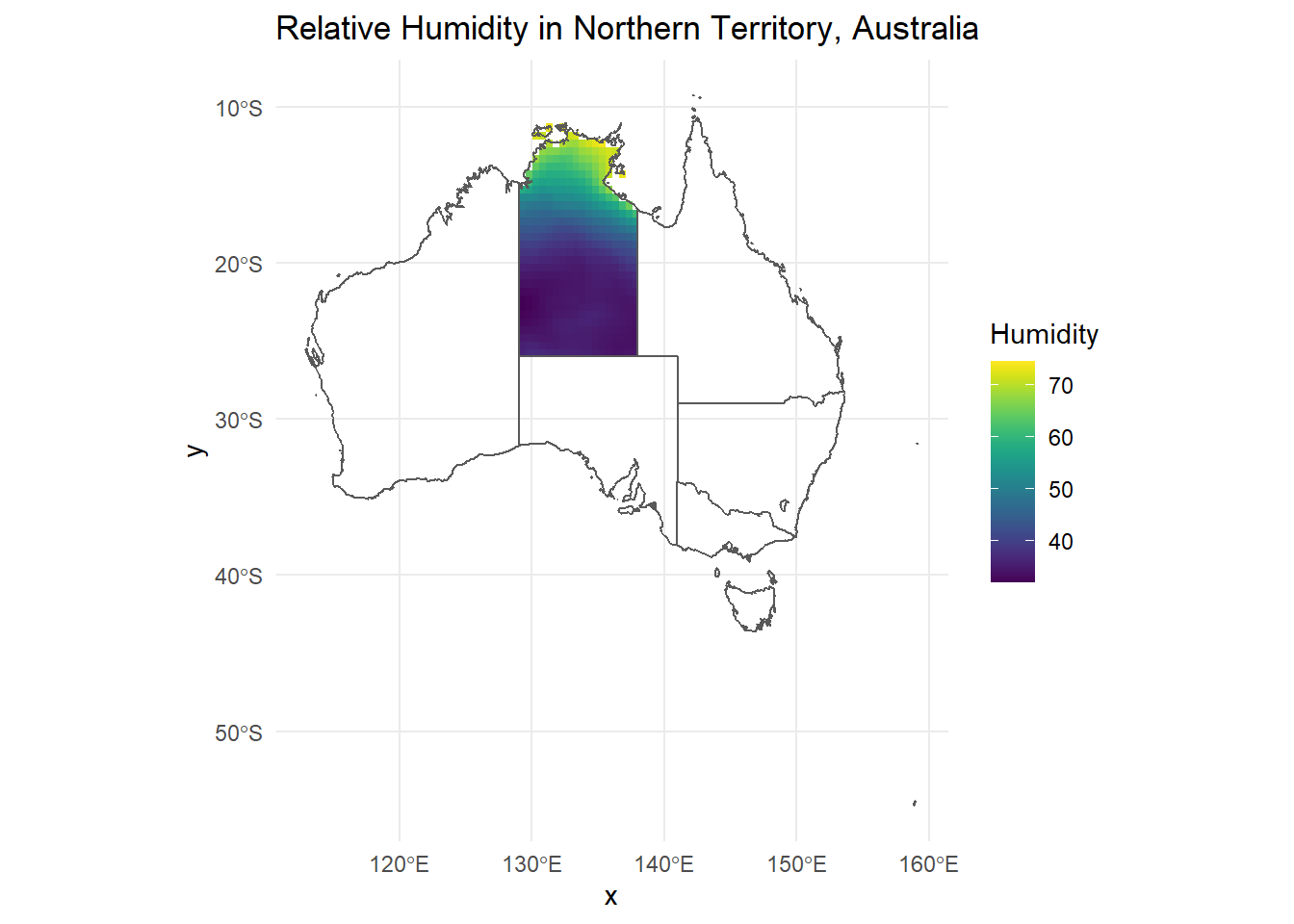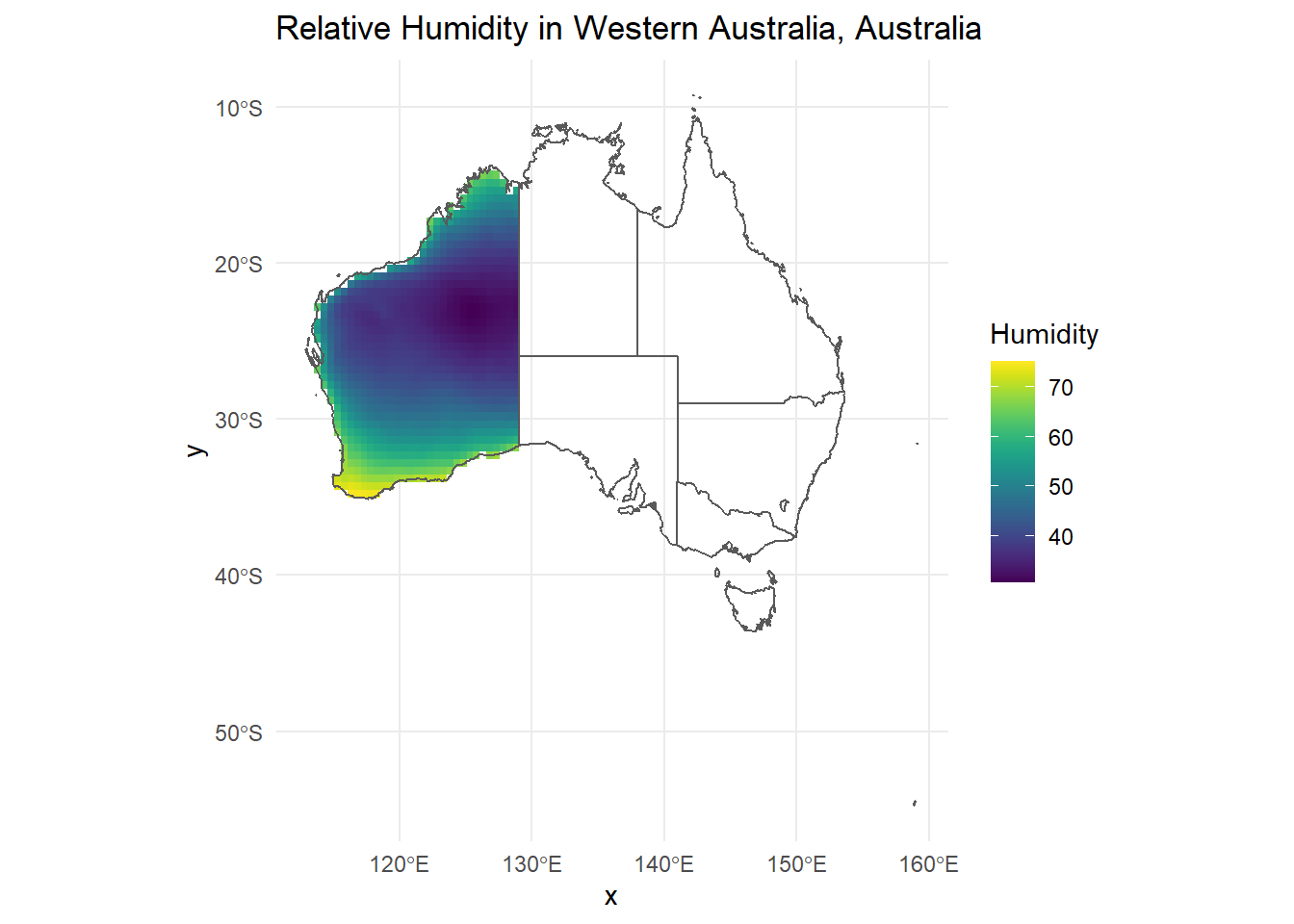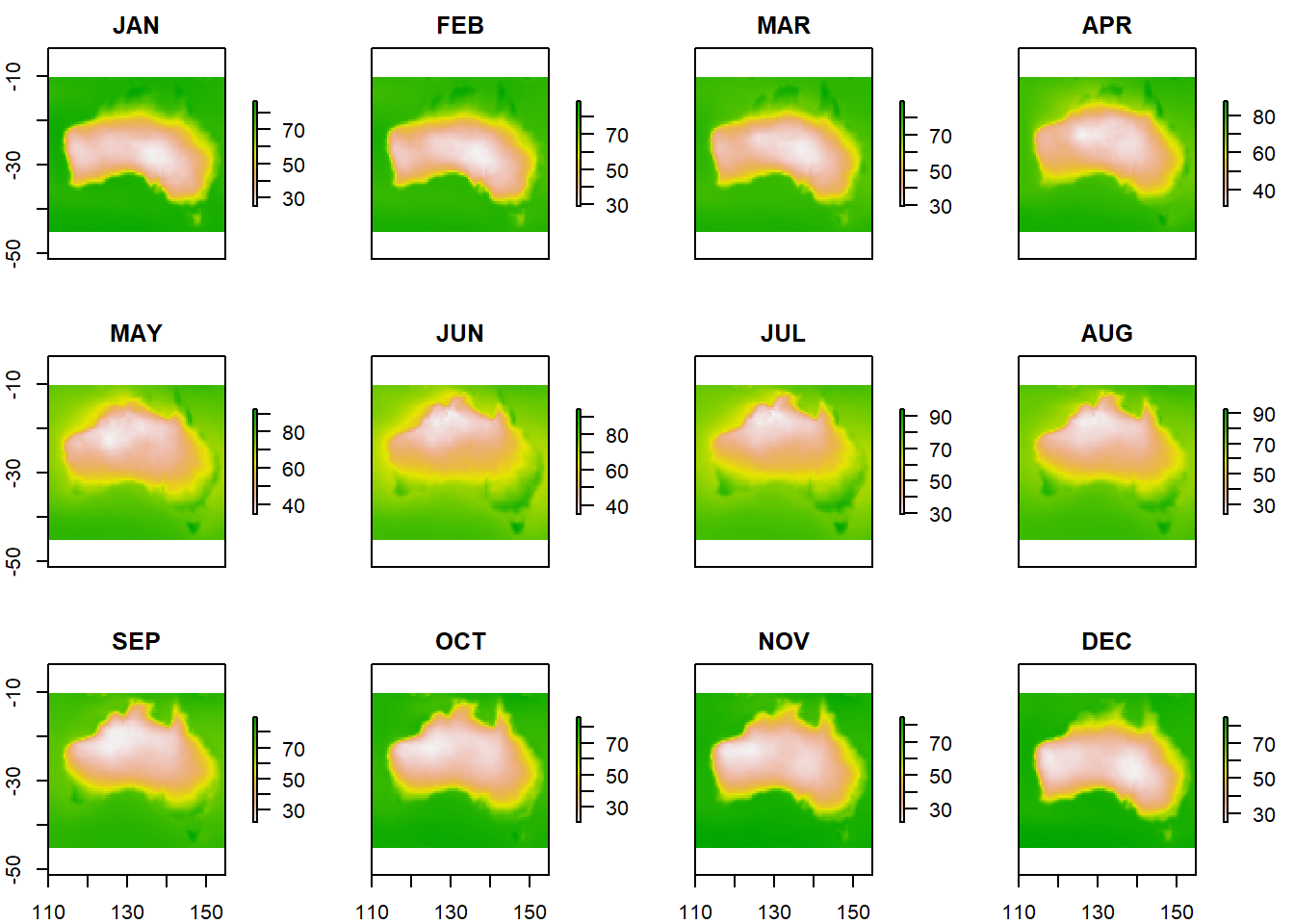Data set description: Humidity data worldwide and in Australia Source: NASA POWER: The POWER Project Details on the retrieved data: Monthly and yearly relative humidity in Northern Territory and Western Australia (Australia). Spatial and temporal resolution: Hourly, daily, monthly, or yearly data for regions defined by the lonlat parameter. Each cell corresponds to 1/2 x 1/2 degree.

## Downloading humidity data with nasapower

The nasapower package aims at making it quick and easy to automate downloading NASA POWER (NASA Prediction of Worldwide Energy Resource) global meteorology, surface solar energy and climatology data.

Here, we will use the nasapower package to retrieve the relative humidity data for specific countries or for the world.

We have also used the nasapower package to retrieve rainfall data here. The rainfall tutorial includes an introduction on the nasapower package and how its functions work.

## Installing nasapower package

We can install the package from CRAN and load it as follows.

install.packages("nasapower")
library(nasapower)

## Using get_power() to fetch data

First let us have a look at how to get the daily data for humidity in agriculture. This can be done using the get_power() function.

### Fetching daily data for single point

We use get_power() function arguments pars = "RH2M" which means relative humidity at 2 meters, temporal_api = "DAILY", and longlat equal to a single location.

data_RH <- get_power(
community = "AG",
lonlat = c(134.489563, -25.734968),
dates = c("2010-09-23", "2010-12-23"),
temporal_api = "DAILY",
pars = "RH2M"
)

data_RH %>% datatable(extensions = c("Scroller", "FixedColumns"), options = list(
deferRender = TRUE,
scrollY = 350,
scrollX = 350,
dom = "t",
scroller = TRUE,
fixedColumns = list(leftColumns = 3)
))

### Fetching daily data for an area

daily_humidity <- get_power(
community = "AG",
lonlat = c(150, -30, 155, -25),
pars = "RH2M",
dates = c("2004-09-19", "2004-09-29"),
temporal_api = "DAILY"
)

daily_humidity %>% datatable(extensions = c("Scroller", "FixedColumns"), options = list(
deferRender = TRUE,
scrollY = 350,
scrollX = 350,
dom = "t",
scroller = TRUE,
fixedColumns = list(leftColumns = 3)
))

### Fetching climatology data

For pars = "RH2M", and as in the rainfall tutorial we will focus on Australia territory and set community = "AG" and temporal_api = "CLIMATOLOGY".

flag <- 1
for (i in seq(110, 150, 5)) {
for (j in seq(-40, -10, 5)) {
climate_avg_RH_temp <- get_power(community = "AG",
pars = "RH2M",
lonlat = c(i, (j - 5), (i + 5), j),
temporal_api = "CLIMATOLOGY")
if (flag == 1) {
climate_avg_RH <- climate_avg_RH_temp
flag <- 0
} else{
climate_avg_RH <- rbind(climate_avg_RH, climate_avg_RH_temp)
}
}
}

climate_avg_RH %>% datatable(extensions = c("Scroller", "FixedColumns"), options = list(
deferRender = TRUE,
scrollY = 350,
scrollX = 350,
dom = "t",
scroller = TRUE,
fixedColumns = list(leftColumns = 3)
))

## Creating a map of annual humidity using all data retrieved

library(rnaturalearth)
library(raster)

# Getting world map
map <- ne_countries(country = 'australia', returnclass = "sf")

# Converting data to raster
r <- rasterFromXYZ(climate_avg_RH[, c("LON", "LAT", "ANN")])

# Converting the raster into a data.frame
r_df <- as.data.frame(r, xy = TRUE, na.rm = TRUE)

# Plot
ggplot() +
geom_raster(data = r_df, aes(x = x, y = y, fill = ANN)) +
geom_sf(data = map, inherit.aes = FALSE, fill = NA) +
scale_fill_viridis() +
labs(
title = "Relative humidity",
fill = "Humidity",
subtitle = "Annual relative humidity in Australia"
)## Creating a map of annual humidity using a subset of the data retrieved

library(rnaturalearth)

# Getting map for China
AUS <- ne_states(country = "Australia", returnclass = "sf")

# Getting administrative boundaries for regions
NT <- AUS[AUS$name == "Northern Territory", ] WA <- AUS[AUS$name == "Western Australia", ]

# Converting data to raster
r <- rasterFromXYZ(climate_avg_RH[, c("LON", "LAT", "ANN")])

# Subset values for the region and converting the raster into a data.frame
r_df <- as.data.frame(rr, xy = TRUE, na.rm = TRUE)

ggplot() +
geom_raster(data = r_df, aes(x = x, y = y, fill = ANN)) +
geom_sf(data = AUS, inherit.aes = FALSE, fill = NA) +
scale_fill_viridis() +
theme_minimal() +
labs(title = "Relative Humidity in Northern Territory, Australia", fill = "Humidity")# Subset values for the region and converting the raster into a data.frame
r_df <- as.data.frame(rr, xy = TRUE, na.rm = TRUE)

ggplot() +
geom_raster(data = r_df, aes(x = x, y = y, fill = ANN)) +
geom_sf(data = AUS, inherit.aes = FALSE, fill = NA) +
scale_fill_viridis() +
theme_minimal() +
labs(title = "Relative Humidity in Western Australia, Australia", fill = "Humidity")## Creating maps of monthly humidity

r <- list()
for (k in colnames(climate_avg_RH)[-c(1:3, 16)]) {
r[[k]] <- rasterFromXYZ(climate_avg_RH[, c("LON", "LAT", k)])
}
r <- stack(r)
plot(r)Corrections: If you see mistakes or want to suggest changes, please create an issue on the source repository or submit a pull request
Contributions: If you want to contribute or collaborate on the project, please see the guidelines for collaborating
Reuse: Text and figures are licensed under Creative Commons Attribution CC BY 4.0.